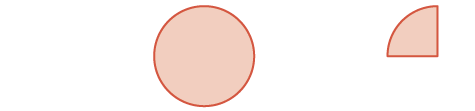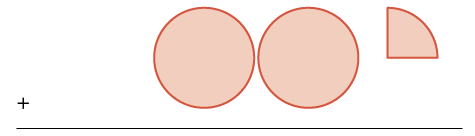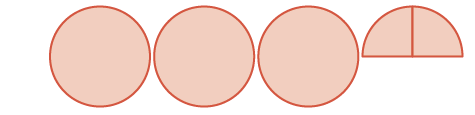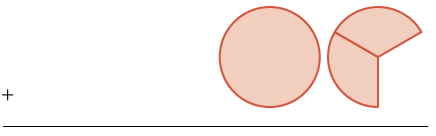## Adding Mixed Numbers With Common Denominators

### Learning Outcomes

• Use a model to add two mixed numbers with like denominators
• Use two different methods to add mixed numbers with like denominators

## Model addition of two mixed numbers with like denominators

So far, we’ve added and subtracted proper and improper fractions, but not mixed numbers. Let’s begin by thinking about addition of mixed numbers using money.

If Ron has $1$ dollar and $1$ quarter, he has $1\Large\frac{1}{4}$ dollars.

If Don has $2$ dollars and $1$ quarter, he has $2\Large\frac{1}{4}$ dollars.

What if Ron and Don put their money together? They would have $3$ dollars and $2$ quarters. They add the dollars and add the quarters. This makes $3\Large\frac{2}{4}$ dollars. Because two quarters is half a dollar, they would have $3$ and a half dollars, or $3\Large\frac{1}{2}$ dollars.

$1\Large\frac{1}{4}$+$2\Large\frac{1}{4}$
$\text{________}$
$3\Large\frac{2}{4}=\normalsize3\Large\frac{1}{2}$

$1\Large\frac{1}{4}+\normalsize2\Large\frac{1}{4}$

We can use fraction circles to model this same example:

 $1\Large\frac{1}{4}+\normalsize2\Large\frac{1}{4}$ Start with $1\Large\frac{1}{4}$ one whole and one $\Large\frac{1}{4}$ pieces$1\Large\frac{1}{4}$ Add $2\Large\frac{1}{4}$ more. two wholes and one $\Large\frac{1}{4}$ pieces$+ 2\Large\frac{1}{4}$ The sum is: three wholes and two $\Large\frac{1}{4}$ ‘s$3\Large\frac{2}{4} = \normalsize 3\Large\frac{1}{2}$

### Example

Model $2\Large\frac{1}{3}+\normalsize1\Large\frac{2}{3}$ and give the sum.

Solution:
We will use fraction circles, whole circles for the whole numbers and $\Large\frac{1}{3}$ pieces for the fractions.

 two wholes and one $\Large\frac{1}{3}$$2\Large\frac{1}{3}$ plus one whole and two $\Large\frac{1}{3}$ s$+ 1\Large\frac{2}{3}$ sum is three wholes and three $\Large\frac{1}{3}$ s$3\Large\frac{3}{3} = 4$

This is the same as $4$ wholes. So, $2\Large\frac{1}{3}+\normalsize1\Large\frac{2}{3}=4$.

### Example

Model $1\Large\frac{3}{5}+\normalsize2\Large\frac{3}{5}$ and give the sum as a mixed number.

### Try It

Model, and give the sum as a mixed number. Draw a picture to illustrate your model.

$2\Large\frac{5}{6}+\normalsize1\Large\frac{5}{6}$

Model, and give the sum as a mixed number. Draw a picture to illustrate your model.
$1\Large\frac{5}{8}+\normalsize1\Large\frac{7}{8}$

## Add mixed numbers with like denominators

Modeling with fraction circles helps illustrate the process for adding mixed numbers: We add the whole numbers and add the fractions, and then we simplify the result, if possible.

### Add mixed numbers with a common denominator

Step 1. Add the whole numbers.
Step 3. Simplify, if possible.

### Example

Add: $3\Large\frac{4}{9}+\normalsize2\Large\frac{2}{9}$

Solution:

 $3\Large\frac{4}{9}+\normalsize2\Large\frac{2}{9}$ Add the whole numbers. $\color{red}{3} \Large\frac{4}{9}$ $\Large\frac{+\color{red}{2}\frac{2}{9}}{\color{red}{5}}$ Add the fractions. $3\color{red}{ \Large\frac{4}{9}}$ $\Large\frac{+2\color{red}{ \Large\frac{2}{9}}}{5\color{red}{ \Large\frac{6}{9}}}$ Simplify the fraction. $\color{red}{5\Large\frac{6}{9}}=5\Large\frac{2}{3}$

### Try It

In the example above, the sum of the fractions was a proper fraction. Now we will work through an example where the sum is an improper fraction.

### Example

Find the sum: $9\Large\frac{5}{9}+\normalsize5\Large\frac{7}{9}$

### Try It

In the following video we show another example of how to add two mixed numbers.

An alternate method for adding mixed numbers is to convert the mixed numbers to improper fractions and then add the improper fractions. This method is usually written horizontally.

### Example

Add by converting the mixed numbers to improper fractions: $3\Large\frac{7}{8}+4\Large\frac{3}{8}$.

### Try It

The table below compares the two methods of addition, using the expression $3\Large\frac{2}{5}+\normalsize6\Large\frac{4}{5}$ as an example. Which way do you prefer?

Mixed Numbers Improper Fractions
$\begin{array}{}\\ \\ \hfill 3\frac{2}{5}\hfill \\ \hfill \frac{+6\frac{4}{5}}{9\frac{6}{5}}\hfill \\ \hfill 9+\frac{6}{5}\hfill \\ \hfill 9+1\frac{1}{5}\hfill \\ \hfill 10\frac{1}{5}\hfill \end{array}$ $\begin{array}{}\\ \hfill 3\frac{2}{5}+6\frac{4}{5}\hfill \\ \hfill \frac{17}{5}+\frac{34}{5}\hfill \\ \hfill \frac{51}{5}\hfill \\ \hfill 10\frac{1}{5}\hfill \end{array}$